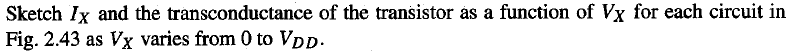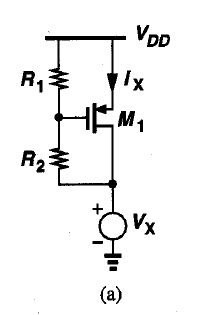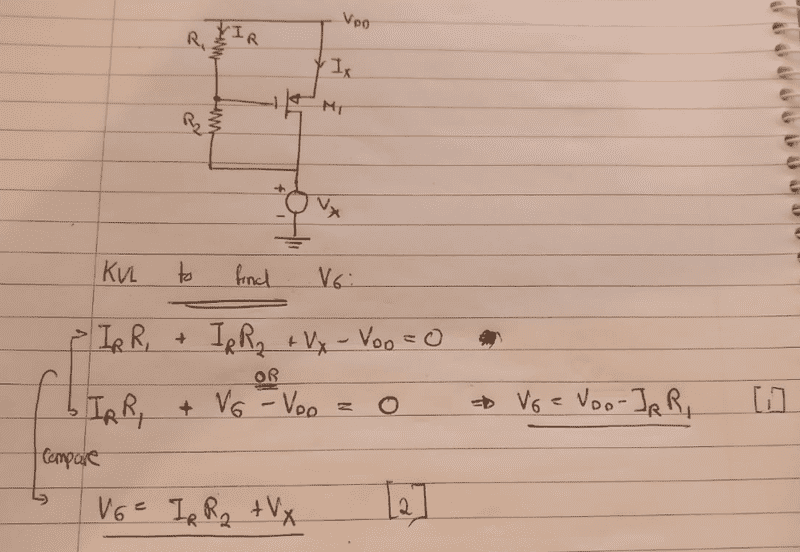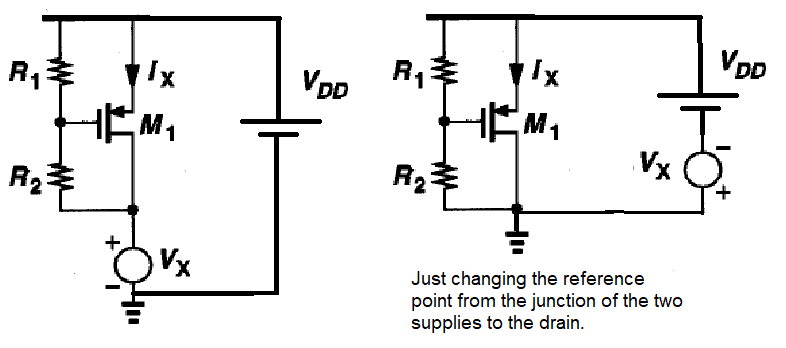# MOSFET KVL Confusion: Finding Vg | Homework Solutions

• CoolDude420
In summary, this person is trying to solve a homework equation but is confused about how voltages are connected in series. They need to rearrange the equations to get a formula for IR. They also mention that if they need to solve for Vg, they can just use Vx in terms of Vds to get the correct answer.f

## Homework Statement## The Attempt at a Solution

My first attempt was to figure out what region the PMOS is operating in so that I could decide what equation to use - so I tried to find Vgs. Vgs = Vg - Vs. Vs is of course just Vdd but for Vg I did a KVL loop as below:My only question is. Now what do I do? How do I get rid of the I_2 in my equations for Vg??

#### Attachments

Since you don't know ANY of the values, you can only get expressions for any of them in terms of the others.
You can rearrange your two equations !,2 to give a formula for IR, but you could have written that down as a starting equation using Ohm's law.

Since you don't have values for R1 and R2, I think you'd be better off starting from the other end. Sketch the sort of curve you would get, then write expressions for the significant points.

If you notice Vx and VDD are in series, you could set the reference point for voltage to be the junction of drain and R2, then the source voltage just varies from VDD to zero. Vg is always a fixed fraction of Vds.

Since you don't know ANY of the values, you can only get expressions for any of them in terms of the others.
You can rearrange your two equations !,2 to give a formula for IR, but you could have written that down as a starting equation using Ohm's law.

Since you don't have values for R1 and R2, I think you'd be better off starting from the other end. Sketch the sort of curve you would get, then write expressions for the significant points.

If you notice Vx and VDD are in series, you could set the reference point for voltage to be the junction of drain and R2, then the source voltage just varies from VDD to zero. Vg is always a fixed fraction of Vds.
How exactly are Vdd and Vx in series? I mean they can form 1 loop but how are they in series if there's a transistor drain connected to Vx? I'm just quite confused. Usually KVL get's me out of situations like these.

You can't see they're in series, because your cct (a) is incomplete. It doesn't show where VDD comes from. I just used a cell to symbolise it and complete the cct.
The Earth point is purely arbitrary - simply a reference point for voltages. You could put it anywhere. I just wanted the complete cct to look like the diagrams in the textbooks.After you've calculated values in the righthand cct, you can change them back to the LH circuit values by simply adding Vx.

The great thing about this cct is that we can assume Ig=0, so Vg is easy to calculate.in terms of Vds. It doesn't matter what IR is. It will always be the same fraction of Vds. I just would never introduce IR into the plot. It's a red herring.

Edit: Just noticed I missed the g subscript in," we can assume Ig=0, "

#### Attachments

Last edited:
You can't see they're in series, because your cct (a) is incomplete. It doesn't show where VDD comes from. I just used a cell to symbolise it and complete the cct.
The Earth point is purely arbitrary - simply a reference point for voltages. You could put it anywhere. I just wanted the complete cct to look like the diagrams in the textbooks.
View attachment 231024
After you've calculated values in the righthand cct, you can change them back to the LH circuit values by simply adding Vx.

The great thing about this cct is that we can assume I=0, so Vg is easy to calculate.in terms of Vds. It doesn't matter what IR is. It will always be the same fraction of Vds. I just would never introduce IR into the plot. It's a red herring.
Ah I see. I'll try that now.